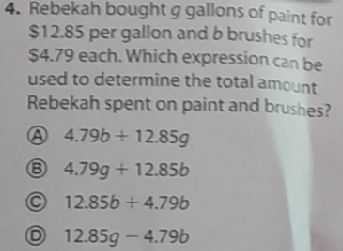### ¿Todavía tienes preguntas de matemáticas?

Pregunte a nuestros tutores expertos
Algebra
Pregunta4. Rebekah bought $$g$$ gallons of paint for S1 $$2.85$$ per gallon and b brushes for

$$\ 4.79$$ each. Which expression can be used to determine the total amount Rebekah spent on paint and brushes?

(A) $$4.79 b + 12.85 g$$

(8) $$4.79 g + 12.85 b$$

(C) $$12.85 b + 4.79 b$$

(D) $$12.85 g - 4.79 b$$